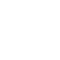# Series Capacitor Voltage – Power System Operation and Control

### Series Capacitor

It is connected in series capacitor to compensate the inductive reactance of line. This reduces the transfer reactance between the buses to which the line is connected. It increases maximum power that can be transmitted and reduces reactive power loss. The reactive power produced by the series capacitor increases with increase in power transfer ; a series capacitor is self regulating in this regard.

### Voltage Regulation

Shunt capacitor banks are connected to H.V. bus or tertiary winding of the main transformer. Shunt capacitors installed at substation to control the voltage within required level. As load varies, voltage at the substation bus and at load bus varies, load power factor is always lagging. A shunt capacitor bank at the substation can raise voltage when the load is high. Shunt capacitor bank can be permanently connected to the bus or can be switched as needed by using predictable load variation.

#### Reducing Power Loss

Compensating the load lagging power factor with the bus connected, shunt capacitor bank improves power factor and reduce the current flowing through the transmission line, transformer, generator. Thereby reducing I²R loss or power loss.

The schematic diagram of a series capacitor installation is shown in image. Under fault conditions, the voltage across the capacitor rises, and unlike a shunt capacitor, series capacitor experiences many times its rated voltage due to fault currents. A zinc oxide varistor in parallel with the capacitor may be adequate to limit this voltage. For locations with high fault currents a parallel fast acting triggered gap is introduced which operates for more severe faults. When the spark gap triggers it is followed by closure of the bypass breaker. The drainage reactor limits the frequency and magnitude of the current through the capacitor when the gap sparks.

The voltage drop across the line is,
IR cos ∅r + I (XL-Xc) sin ∅r,

It is clear from the vector diagram shown in image that the voltage drop produced by an inductive load can be reduced particularly when the line has a high X/R ratio. In practice Xc may be so chosen that the factor (XL – Xc) sin ∅r, becomes negative and numerically equal to R cos ∅r, so that the voltage drop becomes zero. The ratio Xc/XL expressed as a percentage is usually referred to as the percentage compensation.

If I is the full load current, and Xc is the capacitive reactance of the series capacitor, then the drop across the capacitor is I Xc and the VAR rating is I² Xc. The voltage boost produced by the series capacitor is,
ΔV = I Xc sin ∅r

### Drawbacks of Series Capacitor

1. High over-voltage is produced across the capacitor terminals under short circuit conditions. Therefore, very high protective equipment is used. Spark gap.
2. The drop across the capacitor is I Xc where l, is the fault current of the order of 20 times the full load current under certain circuit conditions.
Reactive power supplied by series capacitors is proportional to the square of line current and independent of line voltage.

Location – The locations of series capacitors are:

• Midpoint of the line.
• Line terminals.
• 1/3 or 1/4 th points of the line.

### Problems associated with Series Capacitors

1. Locking of synchronous motor during starting.
2. Hunting of synchronous motor at light load due to high R/X ratio.
3. Ferro resonance occurs between transformers and series capacitors which produces harmonic over voltages.

Series capacitors are used:

• To improve voltage regulation of distribution and industrial feeders.
• To reduce light flicker problems.
• To improve system stability.

#### Applications

The applications of series capacitors are:

1. Voltage rise due to reactive current.
2. By passing the capacitor during faults and reinsertion after fault clearing.